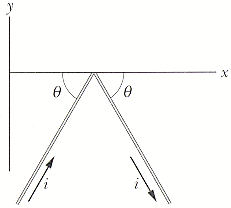Question# The bent wire shown in Fig. lies in a uniform magnetic field.

Other
ANSWEREDThe bent wire shown in Fig. lies in a uniform magnetic field. Each straight section is 2.0 m long and makes an angle of $$\displaystyle={57}^{\circ}$$ with the x axis, and the wire carries a current of 2.0 A.(a) What is the net magnetic force on the wire in unit-vector notation if the magnetic field is 4.0 T?
(b) What is the net magnetic force on the wire in unit-vector notation if the magnetic field is 4.0 T?